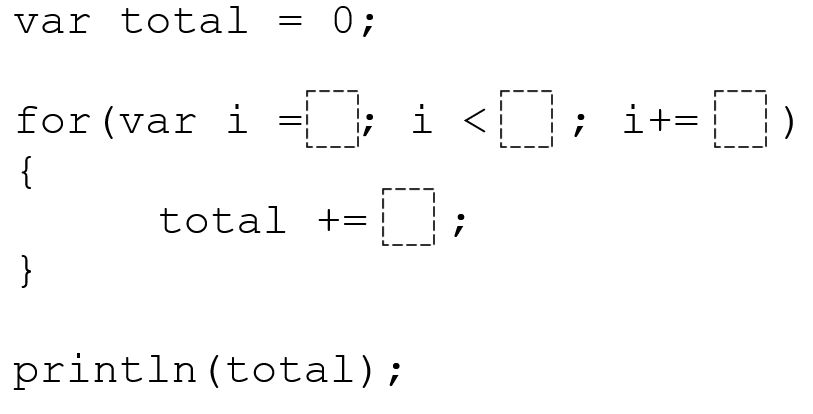# JavaScript – For Loop 1

Directions: In JavaScript, using the digits 1 to 9 at most one time each, place a digit in each box to find then total would have the largest number printed to the screen.### Hint

Be sure to look at how many times the loop iterates, and what it increments by.

56 – var i=2; i<9; i+=1 and total +=8

Source: Jesse Darcy

## JavaScript – For Loops 2

Directions: In JavaScript, using the digits 0 to 9 at most one time each, place …

### One comment

1.56 var(variant) i= 2,i< 9, i+=1 and equal +=8 btw i'm in forth grade